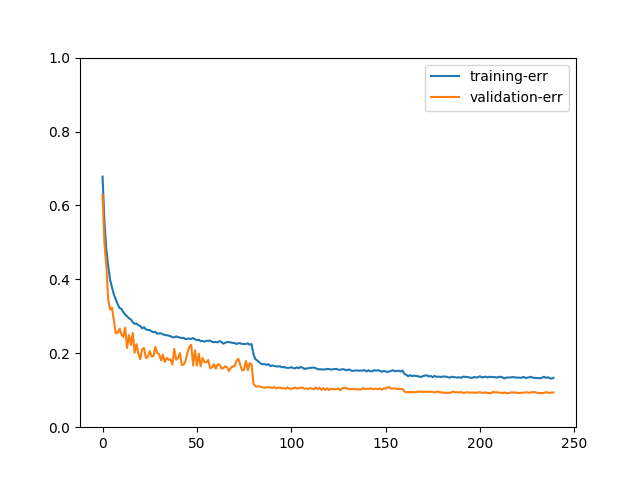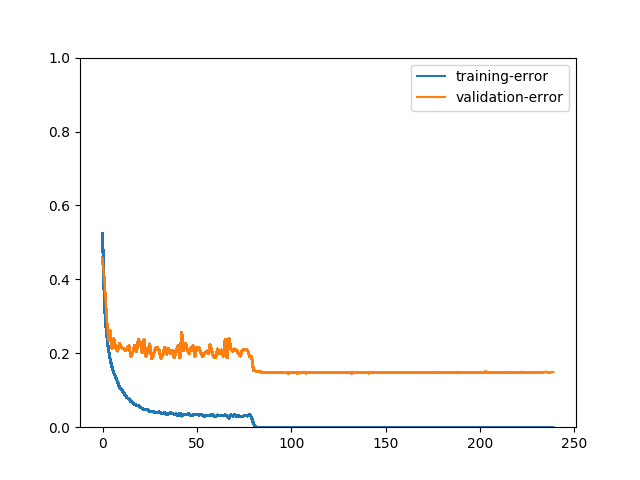# 2. Dive Deep into Training with CIFAR10¶

Hope you enjoyed playing with our demo script. Now, you may be wandering: how exactly was the model trained? In this tutorial, we will focus on answering this question.

## Prerequisites¶

We assume readers have a basic understanding of `Gluon`. If not, we suggest you spend 60 minutes to get started with the Gluon Crash Course .

As we all know, training deep neural networks on GPUs is way faster than training on CPU. In the previous tutorials, we used CPU because classifying a single image is a relatively easy task. However, since we are about to train a model, it is strongly recommended to use a machine with GPU(s).

Note

The rest of the tutorial walks you through the details of `CIFAR10` training. If you want a quick start without knowing the details, try downloading this script and start training with just one command.

`Download train_cifar10.py`

Here’s a sample command with recommended parameters:

```python train_cifar10.py --num-epochs 240 --mode hybrid --num-gpus 1 -j 8 --batch-size 128            --wd 0.0001 --lr 0.1 --lr-decay 0.1 --lr-decay-epoch 80,160 --model cifar_resnet20_v1
```

## Network Structure¶

First, let’s import the necessary libraries into python.

```from __future__ import division

import argparse, time, logging, random, math

import numpy as np
import mxnet as mx

from mxnet import gluon, nd
from mxnet import autograd as ag
from mxnet.gluon import nn
from mxnet.gluon.data.vision import transforms

from gluoncv.model_zoo import get_model
from gluoncv.utils import makedirs, TrainingHistory
from gluoncv.data import transforms as gcv_transforms
```

There are numerous structures for convolutional neural networks. Here we pick a simple yet well-performing structure, `cifar_resnet20_v1`, for the tutorial.

```# number of GPUs to use
num_gpus = 1
ctx = [mx.gpu(i) for i in range(num_gpus)]

# Get the model CIFAR_ResNet20_v1, with 10 output classes, without pre-trained weights
net = get_model('cifar_resnet20_v1', classes=10)
net.initialize(mx.init.Xavier(), ctx = ctx)
```

## Data Augmentation and Data Loader¶

Data augmentation is a common technique used for training. It is base on the assumption that, for the same object, photos under different composition, lighting condition, or color should all yield the same prediction.

Here are photos of the Golden Bridge, taken by many people, at different time from different angles. We can easily tell that they are photos of the same thing.We want to teach this invariance to our model, by playing “augmenting” input image. Our augmentation transforms the image with resizing, cropping, flipping and other techniques.

With `Gluon`, we can create our transform function as following:

```transform_train = transforms.Compose([
# Randomly crop an area and resize it to be 32x32, then pad it to be 40x40
# Randomly flip the image horizontally
transforms.RandomFlipLeftRight(),
# Transpose the image from height*width*num_channels to num_channels*height*width
# and map values from [0, 255] to [0,1]
transforms.ToTensor(),
# Normalize the image with mean and standard deviation calculated across all images
transforms.Normalize([0.4914, 0.4822, 0.4465], [0.2023, 0.1994, 0.2010])
])
```

You may have noticed that most of the operations are randomized. This in effect increases the number of different images the model sees during training. The more data we have, the better our model generalizes over unseen images.

On the other hand, when making prediction, we would like to remove all random operations in order to get a deterministic result. The transform function for prediction is:

```transform_test = transforms.Compose([
transforms.ToTensor(),
transforms.Normalize([0.4914, 0.4822, 0.4465], [0.2023, 0.1994, 0.2010])
])
```

Note that it is important to keep the normalization step, since the model only works well on inputs from the same distribution.

With the transform functions, we can define data loaders for our training and validation datasets.

```# Batch Size for Each GPU
per_device_batch_size = 128
# Number of data loader workers
num_workers = 8
# Calculate effective total batch size
batch_size = per_device_batch_size * num_gpus

# Set train=True for training data
# Set shuffle=True to shuffle the training data
gluon.data.vision.CIFAR10(train=True).transform_first(transform_train),

# Set train=False for validation data
gluon.data.vision.CIFAR10(train=False).transform_first(transform_test),
batch_size=batch_size, shuffle=False, num_workers=num_workers)
```

Out:

```Downloading /root/.mxnet/datasets/cifar10/cifar-10-binary.tar.gz from https://apache-mxnet.s3-accelerate.dualstack.amazonaws.com/gluon/dataset/cifar10/cifar-10-binary.tar.gz...
```

## Optimizer, Loss and Metric¶

Optimizer improves the model during training. Here we use the popular Nesterov accelerated gradient descent algorithm.

```# Learning rate decay factor
lr_decay = 0.1
# Epochs where learning rate decays
lr_decay_epoch = [80, 160, np.inf]

optimizer = 'nag'
# Set parameters
optimizer_params = {'learning_rate': 0.1, 'wd': 0.0001, 'momentum': 0.9}

# Define our trainer for net
trainer = gluon.Trainer(net.collect_params(), optimizer, optimizer_params)
```

In the above code, `lr_decay` and `lr_decay_epoch` are not directly used in `trainer`. One important idea in model training is to gradually decrease learning rate. This means the optimizer takes large steps at the beginning, but step size becomes smaller and smaller in time.

Our plan sets the learning rate to 0.1 at the beginning, then divide it by 10 at the 80-th epoch, then again at the 160-th epoch. We’ll use lr_decay_epoch in the main training loop for this purpose.

In order to optimize our model, we need a loss function. In essence, loss functions compute the difference between predictions and the ground-truth as a measure of model performance. We can then take the gradients of the loss w.r.t. the weights. Gradients points the optimizer to the direction weights should move to improve model performance.

For classification tasks, we usually use softmax cross entropy as the loss function.

```loss_fn = gluon.loss.SoftmaxCrossEntropyLoss()
```

Metrics are similar to loss functions, but they are different in the following aspects:

• Metric is how we evaluate model performance. Each metric is related to a specific task, but independent from the model training process.

• For classification, we usually only use one loss function to train our model, but we can have several metrics for evaluating performance.

• Loss function can be used as a metric, but sometimes its values are hard to interpretate. For instance, the concept “accuracy” is easier to understand than “softmax cross entropy”

For simplicity, we use accuracy as the metric to monitor our training process. Besides, we record metric values, and will print them at the end of training.

```train_metric = mx.metric.Accuracy()
train_history = TrainingHistory(['training-error', 'validation-error'])
```

## Validation¶

Validation dataset provides us a way of monitoring the training process. We have labels for validation data, but they are held out during training. Instead, we use them to evaluate the models performance on unseen data and prevent overfitting.

```def test(ctx, val_data):
metric = mx.metric.Accuracy()
for i, batch in enumerate(val_data):
outputs = [net(X) for X in data]
metric.update(label, outputs)
return metric.get()
```

In order to evaluate performance, we need a metric. Then, we loop through the validation data and predict with our model. We’ll run this function at the end of every epoch to show improvement. over the last epoch.

## Training¶

After all the preparations, we can finally start training! Following is the script.

Note

In order to finish the tutorial quickly, we only train for 3 epochs. In your experiments, we recommend setting `epochs=240`.

```epochs = 3
lr_decay_count = 0

for epoch in range(epochs):
tic = time.time()
train_metric.reset()
train_loss = 0

# Learning rate decay
if epoch == lr_decay_epoch[lr_decay_count]:
trainer.set_learning_rate(trainer.learning_rate*lr_decay)
lr_decay_count += 1

# Loop through each batch of training data
for i, batch in enumerate(train_data):
# Extract data and label

with ag.record():
output = [net(X) for X in data]
loss = [loss_fn(yhat, y) for yhat, y in zip(output, label)]

# Backpropagation
for l in loss:
l.backward()

# Optimize
trainer.step(batch_size)

# Update metrics
train_loss += sum([l.sum().asscalar() for l in loss])
train_metric.update(label, output)

name, acc = train_metric.get()
# Evaluate on Validation data
name, val_acc = test(ctx, val_data)

# Update history and print metrics
train_history.update([1-acc, 1-val_acc])
print('[Epoch %d] train=%f val=%f loss=%f time: %f' %
(epoch, acc, val_acc, train_loss, time.time()-tic))

# We can plot the metric scores with:

train_history.plot()
```Out:

```[Epoch 0] train=0.461779 val=0.605000 loss=72853.881371 time: 15.026766
[Epoch 1] train=0.667067 val=0.701300 loss=46939.770332 time: 14.912694
[Epoch 2] train=0.735437 val=0.739800 loss=37825.300392 time: 14.905922
```

If you trained the model for 240 epochs, the plot may look like:We can better observe the process of model training with plots. For example, one may ask what will happen if there’s no data augmentation:We can see that training error is much lower than validation error. After the model reaches 100% accuracy on training data, it stops improving on validation data. These two plots evidently demonstrates the importance of data augmentation.

After training, we usually want to save it for later use. This is simply done with:

```net.save_parameters('dive_deep_cifar10_resnet20_v2.params')
```

Next time if you need to use it, just run

```net.load_parameters('dive_deep_cifar10_resnet20_v2.params', ctx=ctx)
```

## Next Step¶

This is the end of our adventure with `CIFAR10`, but there are many more datasets and algorithms in computer vision!

If you would like to know how to train a model on a much larger dataset than `CIFAR10`, e.g. ImageNet, please read ImageNet Training.

Or, if you want like to know what can be done with the model you just trained, please read the tutorial on Transfer learning.

Total running time of the script: ( 0 minutes 51.804 seconds)

Gallery generated by Sphinx-Gallery